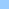# intersect_circle

discussion on how to detect intersections
intersection math gamecircles and rectanges - formula and algorithm ```At 21/42 30/06/99 +0100, darrell wilkins wrote: In lingo it is fairly east to detect if two rectangles intersect using something like: if intersect(rect1,rect2) <> rect(0,0,0,0) then whatever... It is also fairly straight forward to detect if two circles intersect. (if the distance between the two centers < the two radius added together you have a intersect.) But how do you test for the intersect of a circle and a rectange? I'm *not* talking about intersecting sprites thats easy. Rather I want the formula or an algorithm for testing the these sort of intersects. While we are on the subject of algorithms, does anyone know a resource where I can find more on coding techniques. I know there are different sorting algorithms (like bubble sorts etc.) but does anyone know where I might find some other useful routines, ideally with a bit of theory on how they work. (anything that could be useful for games programming would be especially good.) I'm not looking for lingo code neccessarily but rather pseudo code that could be converted into any language. To which Ike Eisenhauer responded: Here is a pseudocode for testing if a circle centered at Xc,Yc with a Radius of R is intersecting a Rectangle centered at Xr,Yr with a height H and a Width W. This assumes computer display coordinate system (ie. X increases to the right and Y increases downward) Step 1. Test intersection of rectangles [Xc-R,Yc-R,Xc+r,Yc+r] and [Xr-W/2,Yr-H/2,Xr+W/2,Yr+H/2] Step 2. If Step 1 is a. False -- Return NotIntersected b. True -- Go to Step 3 Step 3. Calculate a. Value1=(Xr-W/2-Xc)^2 + (Yr-H/2-Yc)^2) b. Value2=(Xr+W/2-Xc)^2 + (Yr-H/2-Yc)^2) c. Value3=(Xr-W/2-Xc)^2 + (Yr+H/2-Yc)^2) d. Value4=(Xr+W/2-Xc)^2 + (Yr+H/2-Yc)^2) Step 4. If all values calculated in Step 3 are > R^2 then Return NotIntersected a. Else Return IntersectionDetected That should get you started. Obviously you need to translate this to Lingo. It's not optimized because I wanted to make it clear not confusing. Any questions on how this works just email me off list. Two good books for algorithms are: A Programmer's Geometery ISBN 0408012420 Intro to Algorithms ISBN 0070131430 Although the last one is nasty deep in math and neither one of them pertained to this problem. And then Darrel Plant said: There are some great articles in recent issues of Game Developer magazine on intersection testing for both 2D and 3D, but here's how I'd start: First test to see whether the rectangle and the bounding box for the circle intersect. If they don't then you know there's no intersection. Pre-generate a series of points for the perimeter of the circle (stored as offsets from the center of the circle, so it can move), and if the bounding box intersect fails, start checking those points for being inside the rectangle. The number of points you generate will obviously slow things down, but 10-30 per circle should suffice, depending on the size of your circles (I did 100000 raw inside () tests in 513ms on a 300 MHz G3). to which Mark Whybird answered: Darrel Plant wrote: > Pre-generate a series of points for the perimeter of the circle (stored as > offsets from the center of the circle, so it can move), and if the bounding > box intersect fails, start checking those points for being inside the > rectangle This is a good solution, but it will fail if the ellipse can completely enclose the rectangle. The deeply mathematical solution would be to find the area of the intersection of the two shapes - if it's > 0, they intersect. Other mathematical solutions that come to mind, like finding any intersections of the line segments and the ellipse fall prey to the same kinds of problems as above - like if the oval encloses the rect, or vice versa - but you could quickly check for these two special cases using the good old rectangle intersects routines (if the intersection rect equals either one of the ones you started with, then one encloses the other.). In fact, you'd do the rect intersects trick first anyway, as a quick way of rejecting cases where they are nowhere near each other, as everyone has pointed out already. Or you could use Danial Plant's very practical solution with the additional test for enclosure with the intersecting rects. I hope this helps, -- Mark Whybird ```Home shock + cgi Bits 'n pieces Director Lingo ShockLets Contact